Next: The Dynamical Phase Up: Laser-driven particle mechanics Previous: Lagrangian and Hamiltonian Formulation   Contents

# Advantage of the Hamiltonian Formulation.

One of the chief virtues of the Lagrangian equations of motion is that they remain invariant under an arbitrary point transformation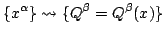Hamilton's equations of motion not only share this virtue but they take it to a higher level: they are invariant under certain more general transformations(5)

which is to say,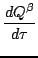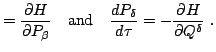(6)

Hereis the transformed superhamiltonian obtained fromwith the help of Eq.(5):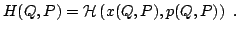Termed canonical, such transformations have the distinguishing property that they leave invariant the representation of the antisymmetric tensorA sufficient condition for a transformation, Eq.(5), to be canonical is that there exist a scalar function of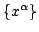and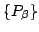,Indeed, lettingand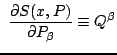(7)

one finds that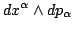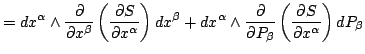zero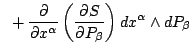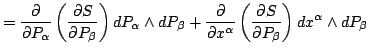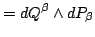(8)

Problem 1: Prove that the representation invariance of Eq.(8) implies the local existence of a scalar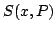whose gradients yield Eq.(7).

Thus the existence of a scalaris both a necessary and a sufficient condition for the invariance expressed by Eq.(8). It also is a sufficient condition for the invariance of Hamilton's equations of motion.

Problem 2: Show that a transformation such as the one given by Eq.(7) transforms the given euations of motion, Eqs.(3)-(4), into the same form, and given by Eq.(6).

Discussion: Taking advantage of the chain rule, letbe a vector tangent to the phasespace trajectory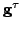relative to the given (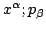) and the new (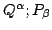) coordinates respectively.

a) Show that

 and(9)

b) Point out why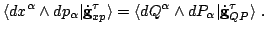c) Show that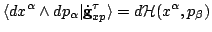implies Hamilton's equations of motion, Eq.(3)-(4).

d) Show that the introduction of the new coordinates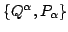into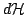,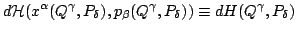yields Eq.(6), Hamilton's equations relative to the new coordinates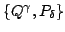.Next: The Dynamical Phase Up: Laser-driven particle mechanics Previous: Lagrangian and Hamiltonian Formulation   Contents
Ulrich Gerlach 2005-11-07